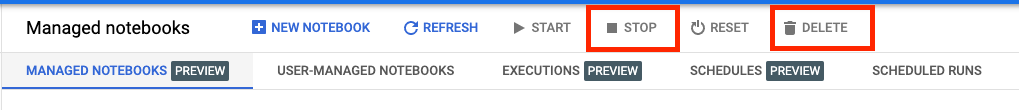# Vertex AI Workbench: Train a TensorFlow model with data from BigQuery

## 1. Overview

In this lab, you'll learn how to use Vertex AI Workbench for data exploration and ML model training.

## What you learn

You'll learn how to:

• Create and configure a Vertex AI Workbench instance
• Use the Vertex AI Workbench BigQuery connector
• Train a model on a Vertex AI Workbench kernel

The total cost to run this lab on Google Cloud is about \$1.

## 2. Intro to Vertex AI

This lab uses the newest AI product offering available on Google Cloud. Vertex AI integrates the ML offerings across Google Cloud into a seamless development experience. Previously, models trained with AutoML and custom models were accessible via separate services. The new offering combines both into a single API, along with other new products. You can also migrate existing projects to Vertex AI.

Vertex AI includes many different products to support end-to-end ML workflows. This lab will focus on Vertex AI Workbench.

Vertex AI Workbench helps users quickly build end-to-end notebook-based workflows through deep integration with data services (like Dataproc, Dataflow, BigQuery, and Dataplex) and Vertex AI. It enables data scientists to connect to GCP data services, analyze datasets, experiment with different modeling techniques, deploy trained models into production, and manage MLOps through the model lifecycle.

## 3. Use Case Overview

In this lab, you'll explore the London Bicycles Hire dataset. This data contains information about bicycle trips from London's public bikesharing program since 2011. You'll start by exploring this dataset in BigQuery through the Vertex AI Workbench BigQuery connector. You'll then load the data into a Jupyter Notebook using pandas and train a TensorFlow model to predict the duration of a cycle trip based on when the trip occurred and how far the person cycled.

This lab makes use of Keras preprocessing layers to transform and prepare the input data for model training. This API allows you to build preprocessing directly into your TensorFlow model graph, reducing the risk of training/serving skew by ensuring that training data and serving data undergo identical transformations. Note that as of TensorFlow 2.6, this API is stable. If you are using an older version of TensorFlow, you'll need to import the experimental symbol.

## 4. Set up your environment

You'll need a Google Cloud Platform project with billing enabled to run this codelab. To create a project, follow the instructions here.

## Step 1: Enable the Compute Engine API

Navigate to Compute Engine and select Enable if it isn't already enabled.

## Step 2: Enable the Vertex AI API

Navigate to the Vertex AI section of your Cloud Console and click Enable Vertex AI API.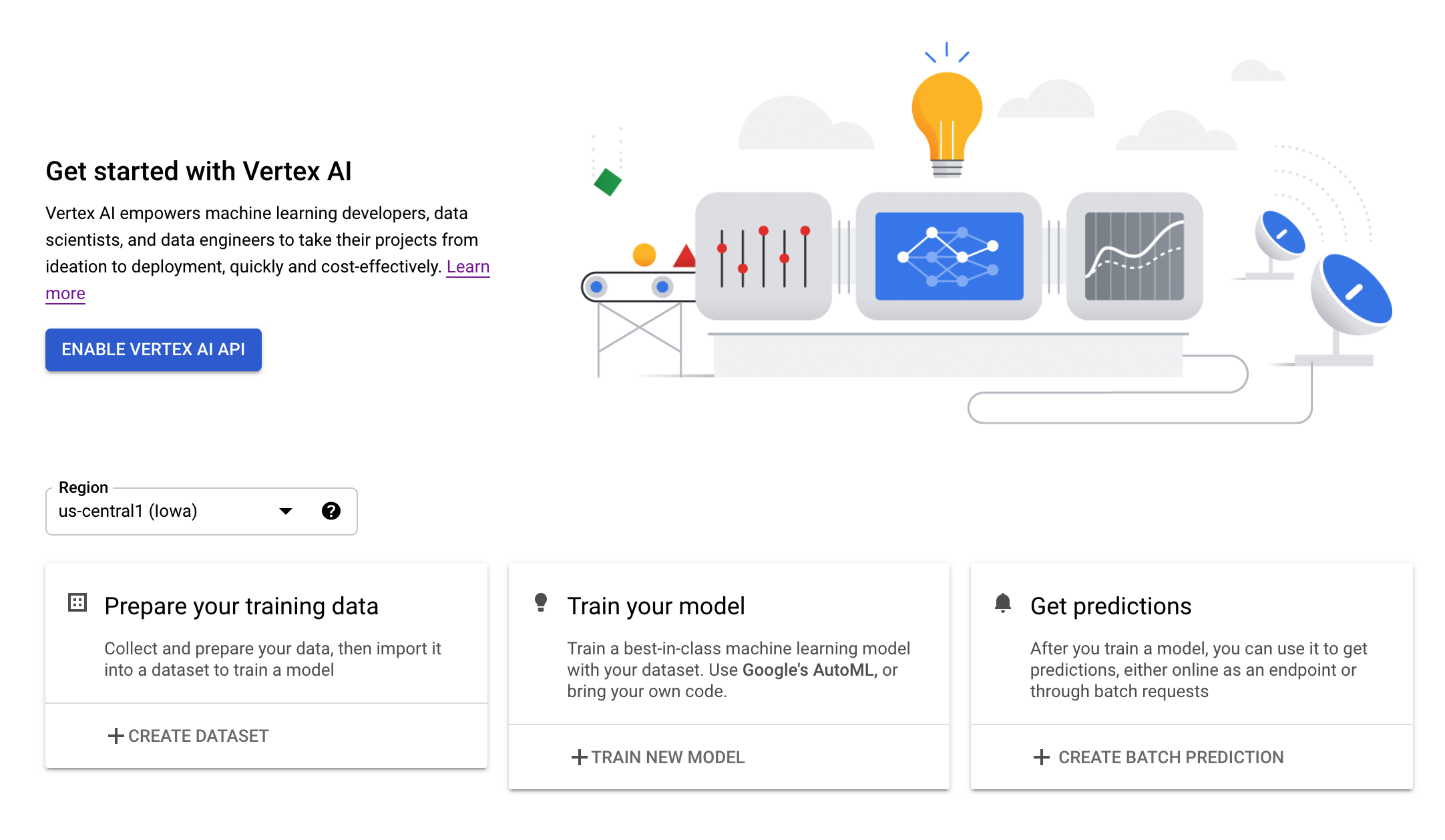## Step 3: Create a Vertex AI Workbench instance

From the Vertex AI section of your Cloud Console, click on Workbench: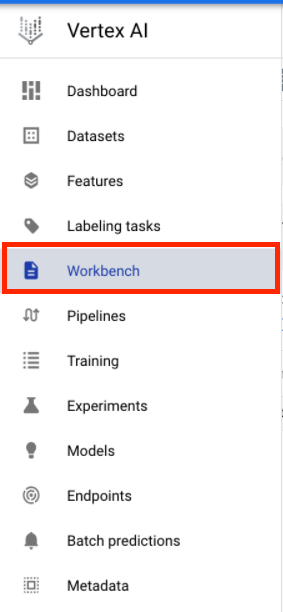Enable the Notebooks API if it isn't already.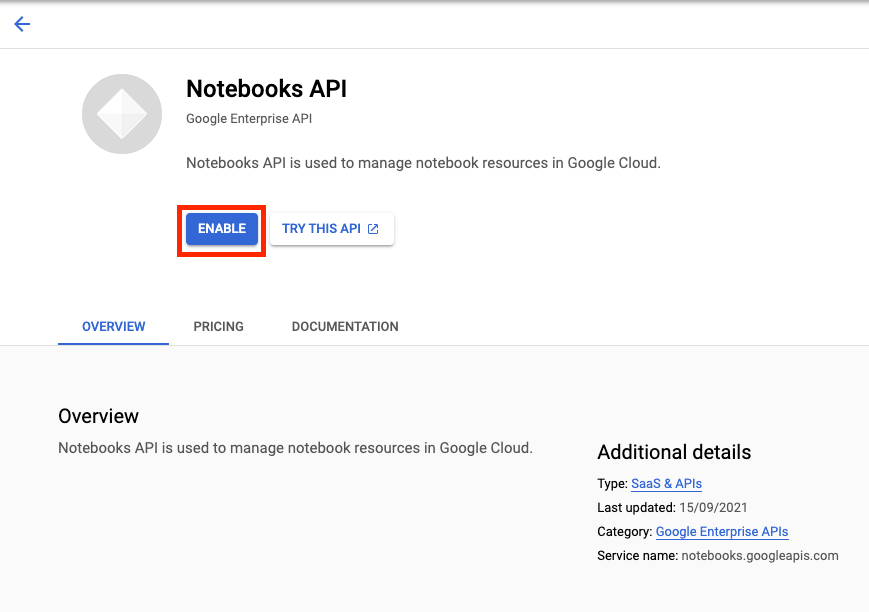Once enabled, click MANAGED NOTEBOOKS: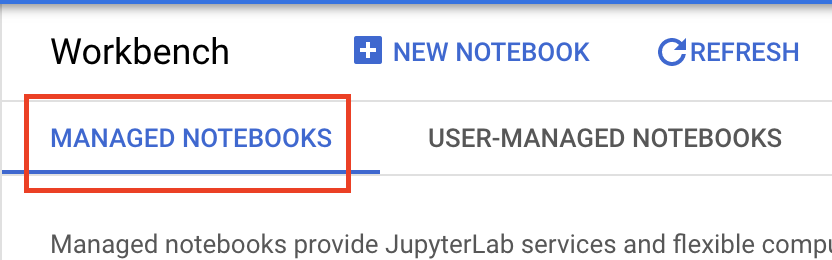Then select NEW NOTEBOOK.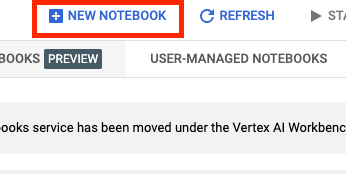Give your notebook a name, and under Permission select Service account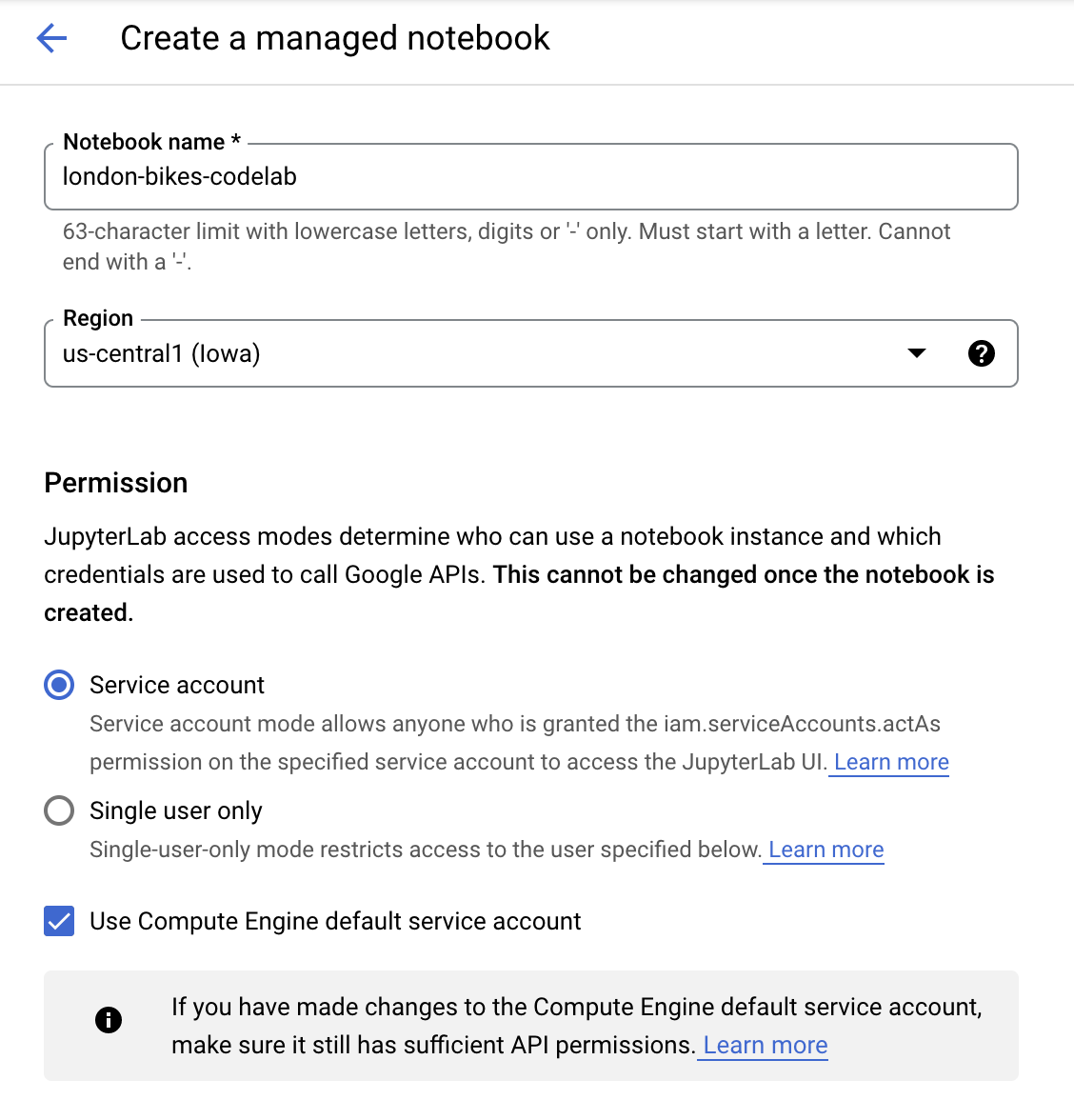Under Security select "Enable terminal" if it is not already enabled.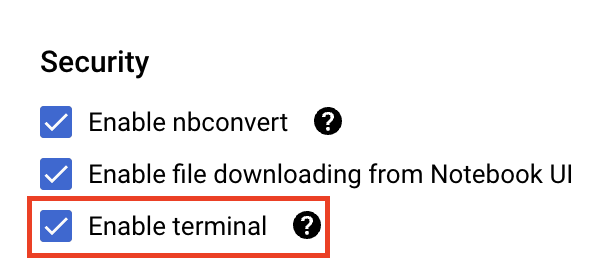You can leave all of the other advanced settings as is.

Next, click Create.

Once the instance has been created, select OPEN JUPYTERLAB.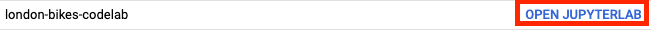## 5. Explore dataset in BigQuery

In the Vertex AI Workbench instance, navigate to the left side and click on the BigQuery in Notebooks connector.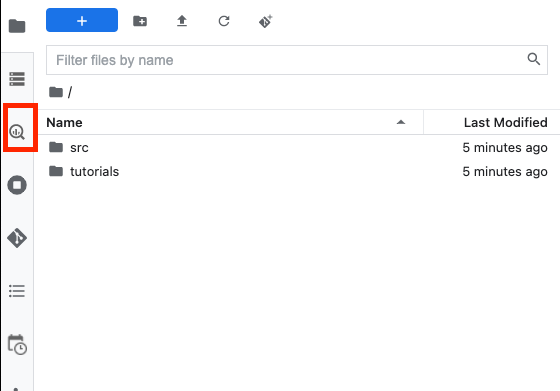The BigQuery connector allows you to easily explore and query BigQuery datasets. In addition to any datasets in your project, you can explore datasets in other projects by clicking the Add Project button.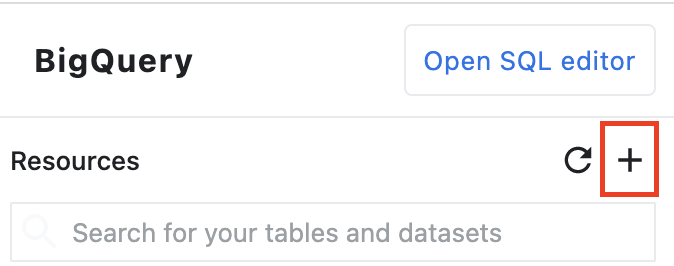For this lab, you'll use data from the BigQuery public datasets. Scroll down until you find the london_bicycles dataset. You'll see that this dataset has two tables, cycle_hire and cycle_stations. Let's explore each of them.First, double click on the cycle_hire table. You'll see that the table opens as a new tab with the table's schema as well as metadata like the number of rows and size.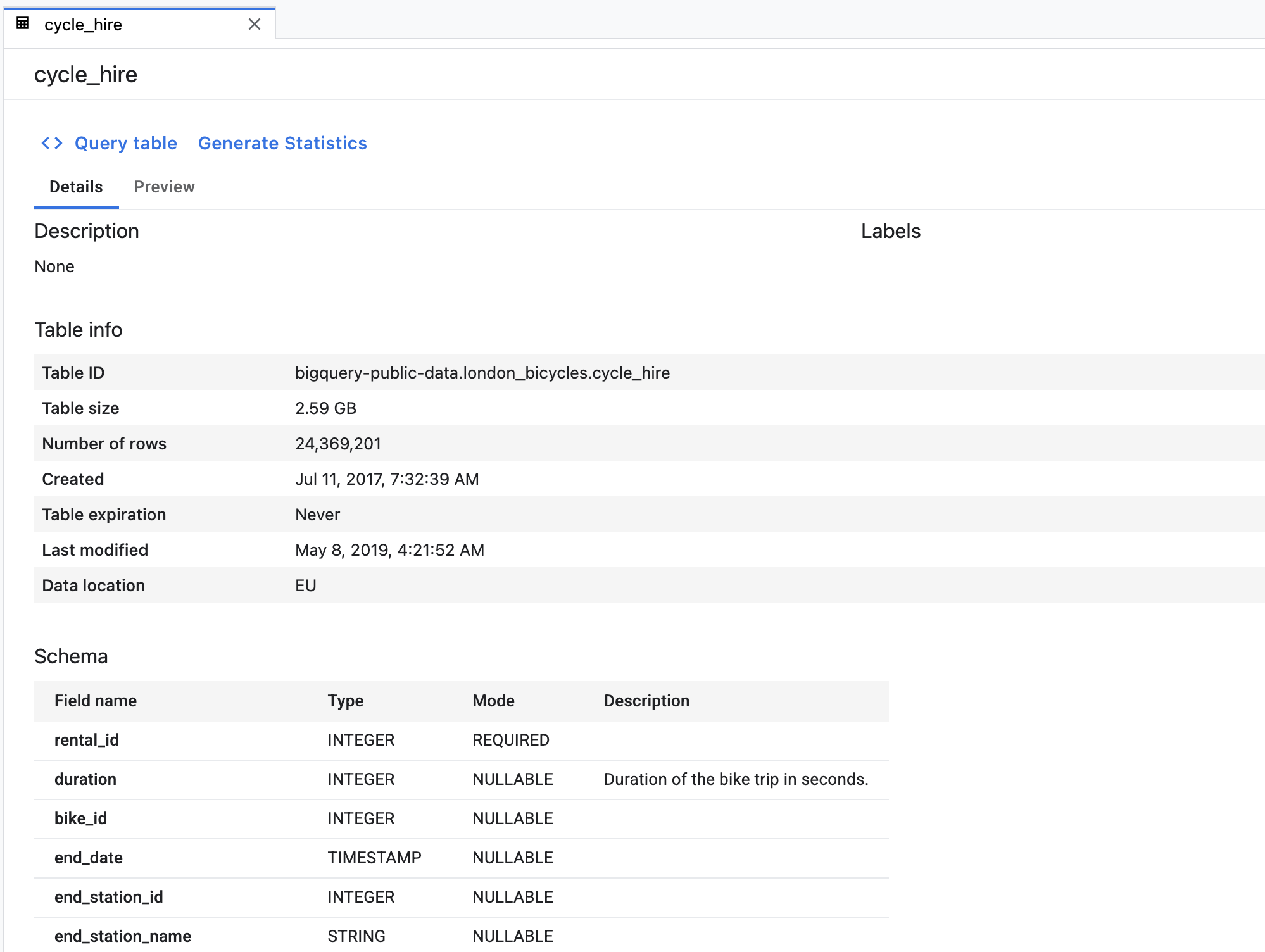If you click on the Preview tab, you'll be able to see a sample of the data. Let's run a simple query to see what the the popular journeys are. First, click the Query table button.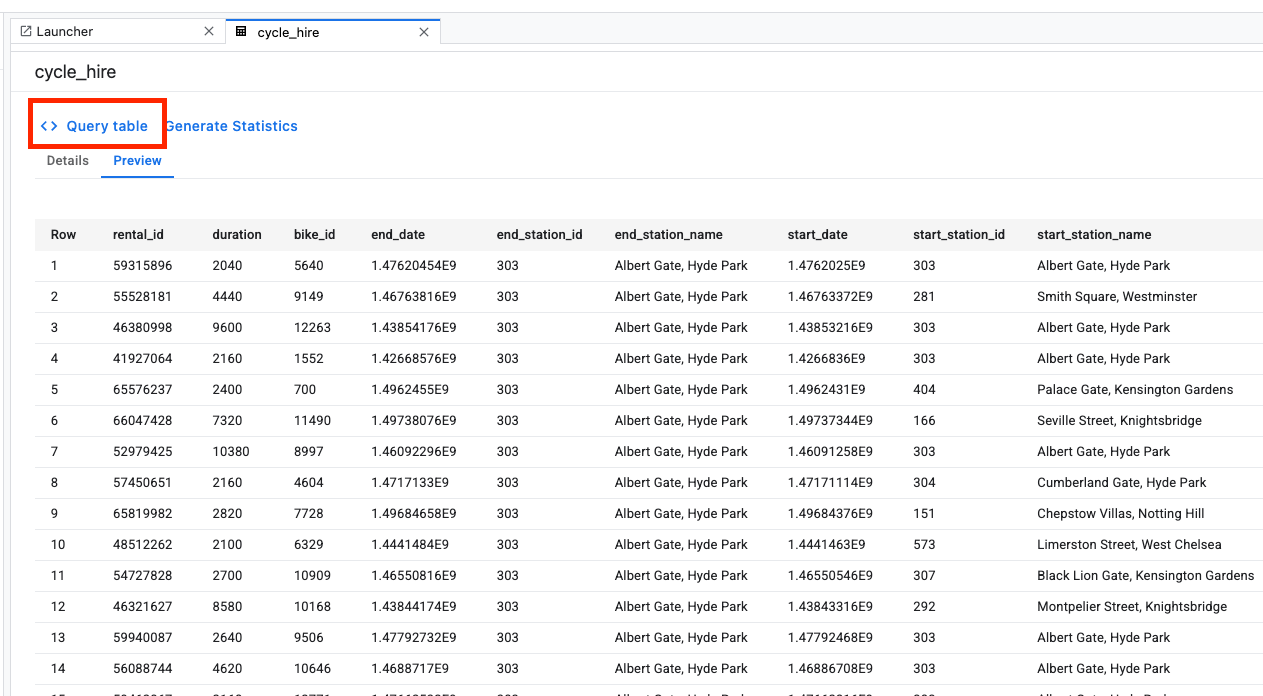Then, paste the following into the SQL editor and click Submit Query.

``````SELECT
start_station_name,
end_station_name,
IF(start_station_name = end_station_name,
TRUE,
FALSE) same_station,
AVG(duration) AS avg_duration,
COUNT(*) AS total_rides
FROM
`bigquery-public-data.london_bicycles.cycle_hire`
GROUP BY
start_station_name,
end_station_name,
same_station
ORDER BY
total_rides DESC
``````

From the query results, you'll see that cycle trips to and from the Hyde Park Corner station were the most popular.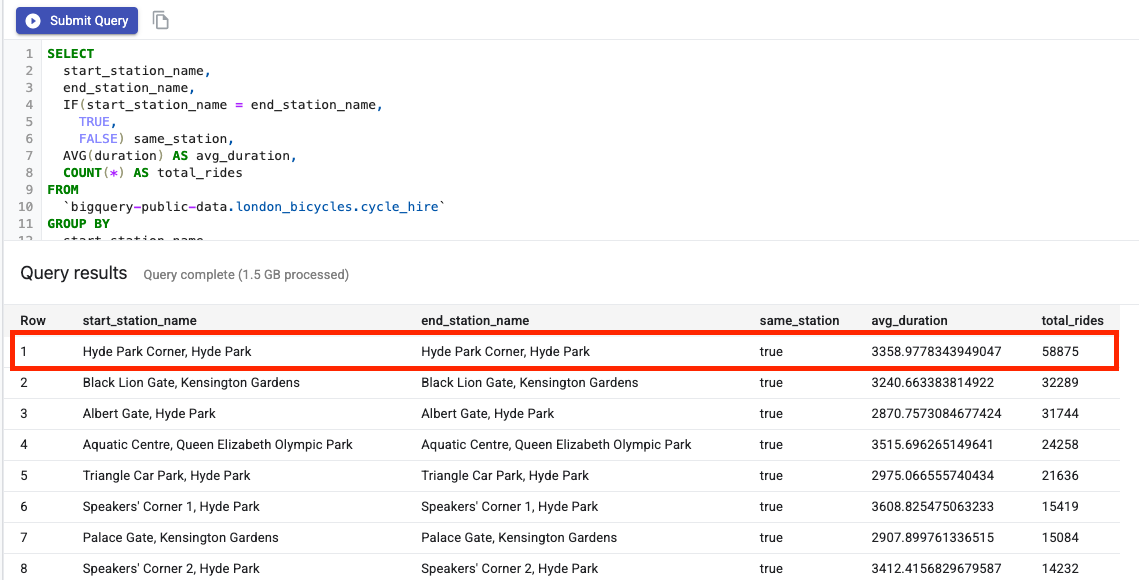Next, double click on the cycle_stations table, which provides information about each station.

We want to join the cycle_hire and cycle_stations tables. The cycle_stations table contains the lat/lon for each station. You'll use this information to estimate the distance traveled on each cycle trip by calculating the distance between the start and end stations.

To do this calculation, you'll use BigQuery geography functions. Specifically, you'll convert each lat/lon string to a ST_GEOGPOINT and use the ST_DISTANCE function to compute the straight line distance in meters between the two points. You'll use this value as a proxy for the distance traveled in each cycle trip.

Copy the following query into your SQL editor and then click Submit Query. Note that there are three tables in the JOIN condition because we need to join the stations table twice to get the lat/lon for both the cycle's starting station and ending station.

``````WITH staging AS (
SELECT
STRUCT(
start_stn.name,
ST_GEOGPOINT(start_stn.longitude, start_stn.latitude) AS POINT,
start_stn.docks_count,
start_stn.install_date
) AS starting,
STRUCT(
end_stn.name,
ST_GEOGPOINT(end_stn.longitude, end_stn.latitude) AS point,
end_stn.docks_count,
end_stn.install_date
) AS ending,
STRUCT(
rental_id,
bike_id,
duration, --seconds
ST_DISTANCE(
ST_GEOGPOINT(start_stn.longitude, start_stn.latitude),
ST_GEOGPOINT(end_stn.longitude, end_stn.latitude)
) AS distance, --meters
start_date,
end_date
) AS bike
FROM `bigquery-public-data.london_bicycles.cycle_stations` AS start_stn
LEFT JOIN `bigquery-public-data.london_bicycles.cycle_hire` as b
ON start_stn.id = b.start_station_id
LEFT JOIN `bigquery-public-data.london_bicycles.cycle_stations` AS end_stn
ON end_stn.id = b.end_station_id
LIMIT 700000)

SELECT * from STAGING

``````

## 6. Train an ML model on a TensorFlow kernel

Vertex AI Workbench has a compute compatibility layer that allows you to launch kernels for TensorFlow, PySpark, R, etc, all from a single notebook instance. In this lab, you'll create a notebook using the TensorFlow kernel.

## Create DataFrame

After the query executes, click on Copy code for DataFrame. This will allow you to paste Python code into a notebook that connects to the BigQuery client and extracts this data as a pandas DataFrame.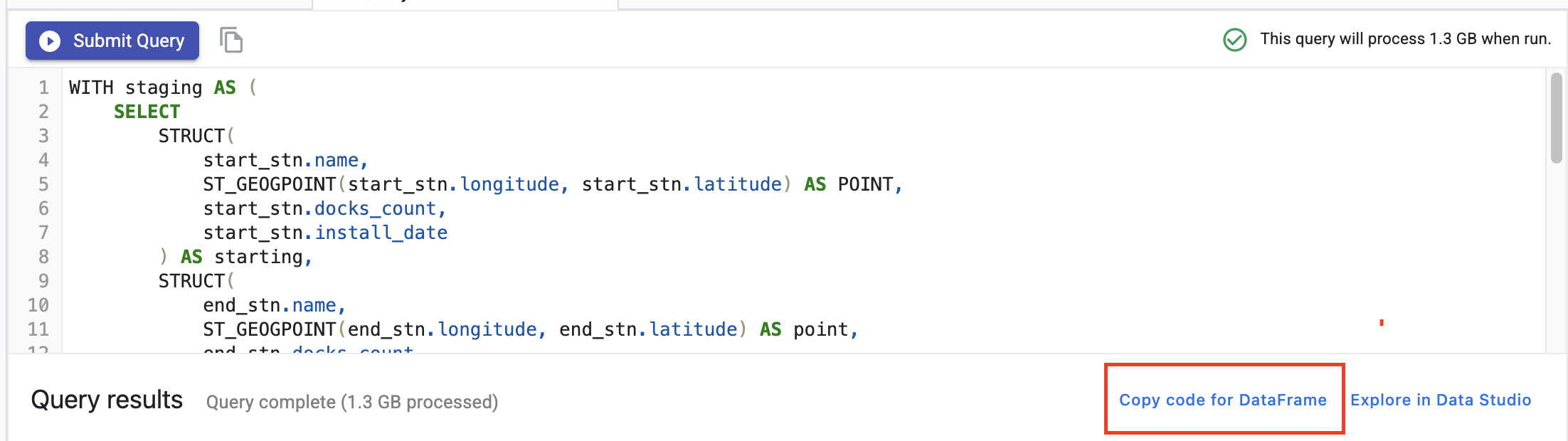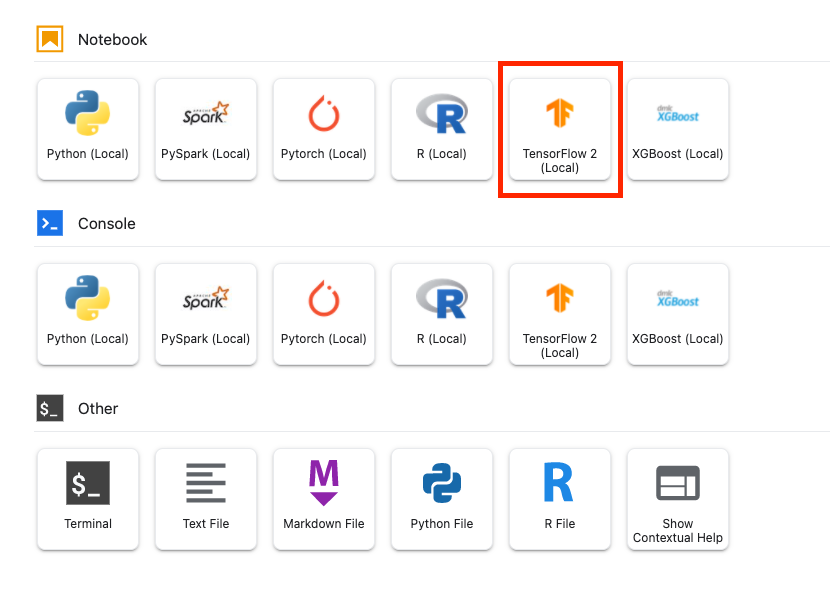In the first cell of the notebook, paste the code copied from the Query Editor. It should look like the following:

``````# The following two lines are only necessary to run once.
# Comment out otherwise for speed-up.
client = Client()

query = """WITH staging AS (
SELECT
STRUCT(
start_stn.name,
ST_GEOGPOINT(start_stn.longitude, start_stn.latitude) AS POINT,
start_stn.docks_count,
start_stn.install_date
) AS starting,
STRUCT(
end_stn.name,
ST_GEOGPOINT(end_stn.longitude, end_stn.latitude) AS point,
end_stn.docks_count,
end_stn.install_date
) AS ending,
STRUCT(
rental_id,
bike_id,
duration, --seconds
ST_DISTANCE(
ST_GEOGPOINT(start_stn.longitude, start_stn.latitude),
ST_GEOGPOINT(end_stn.longitude, end_stn.latitude)
) AS distance, --meters
start_date,
end_date
) AS bike
FROM `bigquery-public-data.london_bicycles.cycle_stations` AS start_stn
LEFT JOIN `bigquery-public-data.london_bicycles.cycle_hire` as b
ON start_stn.id = b.start_station_id
LEFT JOIN `bigquery-public-data.london_bicycles.cycle_stations` AS end_stn
ON end_stn.id = b.end_station_id
LIMIT 700000)

SELECT * from STAGING"""
job = client.query(query)
df = job.to_dataframe()
``````

For the purposes of this lab, we limit the dataset to 700000 to keep training time shorter. But feel free to modify the query and experiment with the entire dataset.

Next, import the necessary libraries.

``````from datetime import datetime
import pandas as pd
import tensorflow as tf
``````

Run the following code to create a reduced dataframe that only contains the columns needed for the ML portion of this exercise.

``````values = df['bike'].values
duration = list(map(lambda a: a['duration'], values))
distance = list(map(lambda a: a['distance'], values))
dates = list(map(lambda a: a['start_date'], values))
data = pd.DataFrame(data={'duration': duration, 'distance': distance, 'start_date':dates})
data = data.dropna()
``````

The start_date column is a Python `datetime`. Instad of using this `datetime` in the model directly, you'll create two new features that indicate the day of the week and the hour of the day that the bike trip occurred.

``````data['weekday'] = data['start_date'].apply(lambda a: a.weekday())
data['hour'] = data['start_date'].apply(lambda a: a.time().hour)
data = data.drop(columns=['start_date'])
``````

Lastly, convert the duration column from seconds to minutes so it's easier to understand

``````data['duration'] = data['duration'].apply(lambda x:float(x / 60))
``````

Examine the first few rows of the formatted DataFrame. For each cycle trip, you now have data on the day of the week and hour of the day that the trip occurred, as well as the distance traveled. From this information, you'll try to predict how long the trip took.

``````data.head()
``````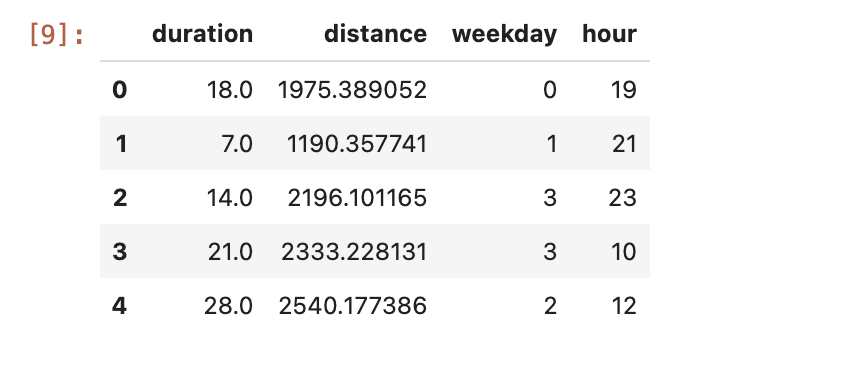Before you can create and train the model, you need to split the data into training and validation sets.

``````# Use 80/20 train/eval split
train_size = int(len(data) * .8)
print ("Train size: %d" % train_size)
print ("Evaluation size: %d" % (len(data) - train_size))

# Split data into train and test sets
train_data = data[:train_size]
val_data = data[train_size:]
``````

## Create TensorFlow model

You'll create a TensorFlow model using the Keras Functional API. To preprocess the input data, you'll make use of the Keras preprocessing layers API.

The following utility function will create a `tf.data.Dataset` out of the pandas Dataframe.

``````def df_to_dataset(dataframe, label, shuffle=True, batch_size=32):
dataframe = dataframe.copy()
labels = dataframe.pop(label)
ds = tf.data.Dataset.from_tensor_slices((dict(dataframe), labels))
if shuffle:
ds = ds.shuffle(buffer_size=len(dataframe))
ds = ds.batch(batch_size)
ds = ds.prefetch(batch_size)
return ds
``````

Use the above function to create two `tf.data.Dataset`s, one for training and one for validation. You might see some warnings, but you can safely ignore them.

``````train_dataset = df_to_dataset(train_data, 'duration')
validation_dataset = df_to_dataset(val_data, 'duration')
``````

You'll use the following preprocessing layers in the model:

• Normalization layer: performs feature-wise normalization of input features.
• IntegerLookup layer: turns integer categorical values into integers indices.
• CategoryEncoding layer: turns integer categorical features into one-hot, multi-hot, or TF-IDF dense representations.

Note that these layers are not trainable. Instead, you set the state of the preprocessing layer by exposing it to training data, via the `adapt()` method.

The following function will create a normalization layer that you can use on the distance feature. You'll set the state prior to fitting the model by using the `adapt()` method on the training data. This will calculate the mean and variance to use for normalization. Later, when you pass the validation dataset to the model, this same mean and variance calculated on the training data will be used to scale the validation data.

``````def get_normalization_layer(name, dataset):
# Create a Normalization layer for our feature.
normalizer = tf.keras.layers.Normalization(axis=None)

# Prepare a Dataset that only yields our feature.
feature_ds = dataset.map(lambda x, y: x[name])

# Learn the statistics of the data.

return normalizer
``````

Similarly, the following function creates a category encoding that you'll use on the hour and weekday features.

``````def get_category_encoding_layer(name, dataset, dtype, max_tokens=None):
index = tf.keras.layers.IntegerLookup(max_tokens=max_tokens)

# Prepare a Dataset that only yields our feature
feature_ds = dataset.map(lambda x, y: x[name])

# Learn the set of possible values and assign them a fixed integer index.

# Create a Discretization for our integer indices.
encoder = tf.keras.layers.CategoryEncoding(num_tokens=index.vocabulary_size())

# Apply one-hot encoding to our indices. The lambda function captures the
# layer so we can use them, or include them in the functional model later.
return lambda feature: encoder(index(feature))
``````

Next, create the preprocessing portion of the model. First, create a `tf.keras.Input` layer for each of the features.

``````# Create a Keras input layer for each feature
numeric_col = tf.keras.Input(shape=(1,), name='distance')
hour_col = tf.keras.Input(shape=(1,), name='hour', dtype='int64')
weekday_col = tf.keras.Input(shape=(1,), name='weekday', dtype='int64')
``````

Then create the normalization and category encoding layers, storing them in a list.

``````all_inputs = []
encoded_features = []

# Pass 'distance' input to normalization layer
normalization_layer = get_normalization_layer('distance', train_dataset)
encoded_numeric_col = normalization_layer(numeric_col)
all_inputs.append(numeric_col)
encoded_features.append(encoded_numeric_col)

# Pass 'hour' input to category encoding layer
encoding_layer = get_category_encoding_layer('hour', train_dataset, dtype='int64')
encoded_hour_col = encoding_layer(hour_col)
all_inputs.append(hour_col)
encoded_features.append(encoded_hour_col)

# Pass 'weekday' input to category encoding layer
encoding_layer = get_category_encoding_layer('weekday', train_dataset, dtype='int64')
encoded_weekday_col = encoding_layer(weekday_col)
all_inputs.append(weekday_col)
encoded_features.append(encoded_weekday_col)

``````

After defining the preprocessing layers, you can define the rest of the model. You'll concatenate all of the input featues, and pass them to a dense layer. The output layer is a single unit since this is a regression problem.

``````all_features = tf.keras.layers.concatenate(encoded_features)
x = tf.keras.layers.Dense(64, activation="relu")(all_features)
output = tf.keras.layers.Dense(1)(x)
model = tf.keras.Model(all_inputs, output)
``````

Lastly, compile the model.

``````model.compile(optimizer = tf.keras.optimizers.Adam(0.001),
loss='mean_squared_logarithmic_error')
``````

Now that you have defined the model, you can visualize the architecture

``````tf.keras.utils.plot_model(model, show_shapes=True, rankdir="LR")
``````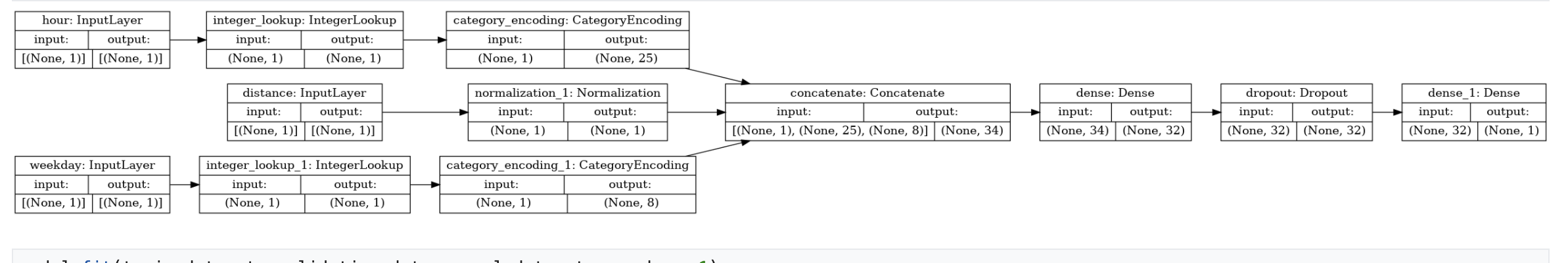Note that this model is fairly complicated for this simple dataset. It is intended for demonstration purposes.

Let's train for 1 epoch just to confirm that the code runs.

``````model.fit(train_dataset, validation_data = validation_dataset, epochs = 1)
``````

## Train model with a GPU

Next, you'll train the model for longer and use the hardware switcher to speed up training. Vertex AI Workbench allows you to change the hardware without shutting down your instance. By adding the GPU only when you need it, you can keep costs lower.

To change the hardware profile, click on the machine type in the top right corner and select Modify hardware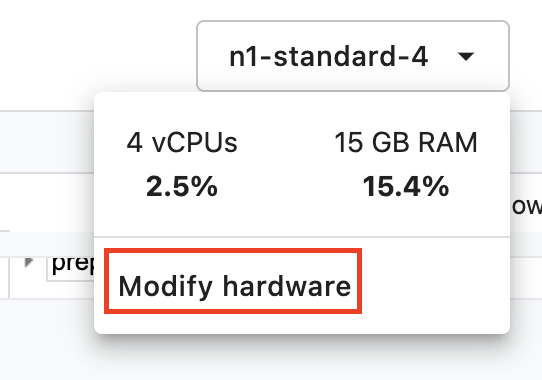Select Attach GPUs and select an NVIDIA T4 Tensor Core GPU.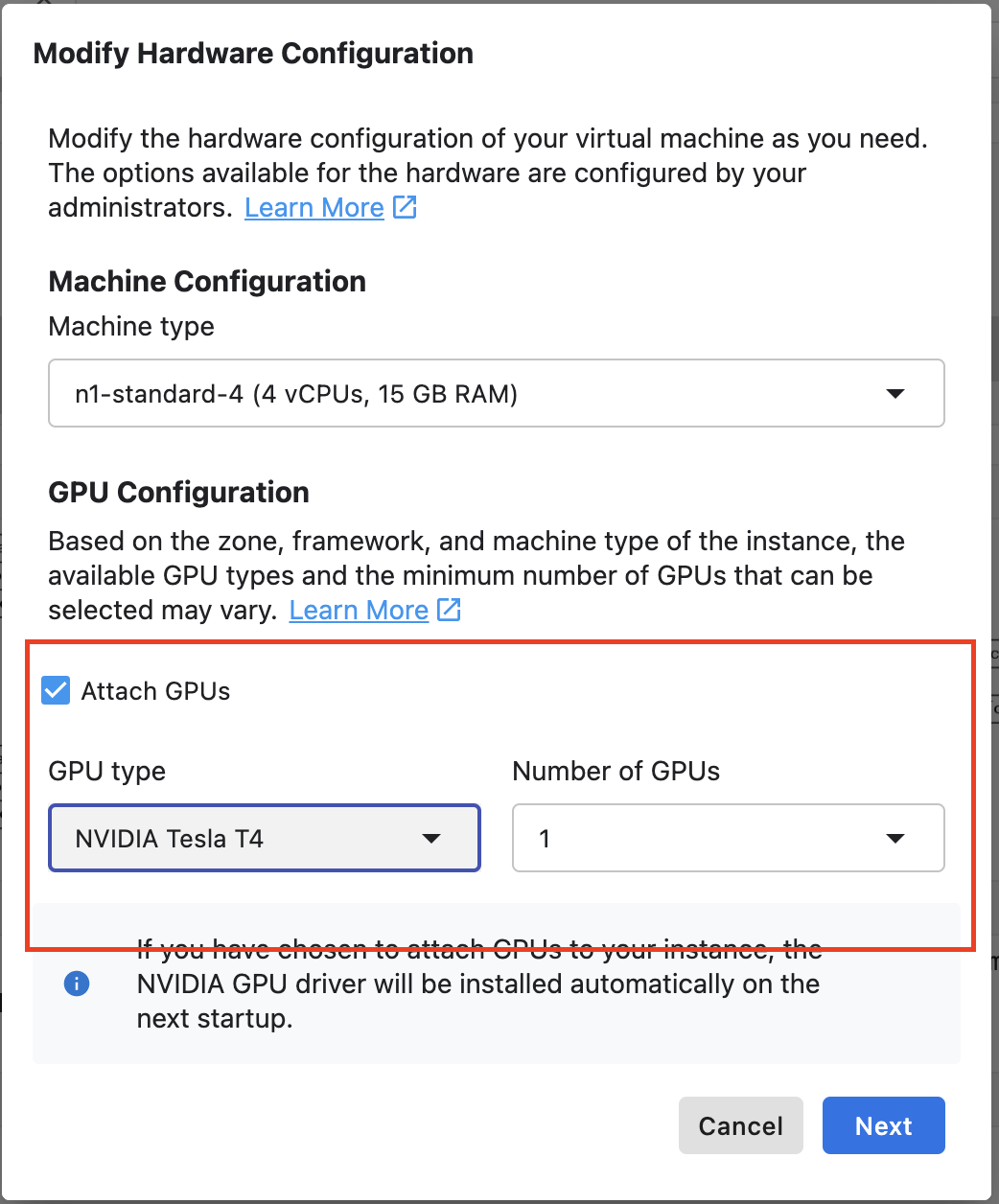It will take around five minutes for the hardware to be configured. Once the process is complete, let's train the model for a little longer. You'll notice that each epoch takes less time now.

``````model.fit(train_dataset, validation_data = validation_dataset, epochs = 5)
``````

🎉 Congratulations! 🎉

You've learned how to use Vertex AI Workbench to:

• Explore data in BigQuery
• Use the BigQuery client to load data into Python
• Train a TensorFlow model with Keras Preprocessing Layers and a GPU